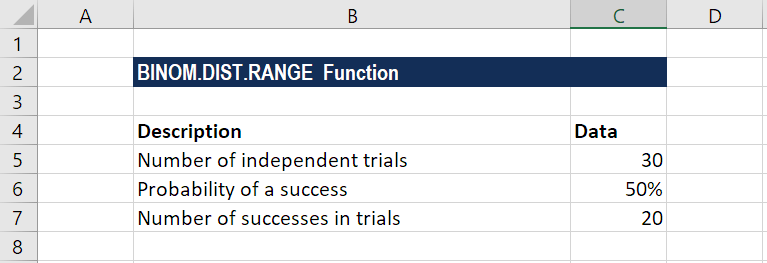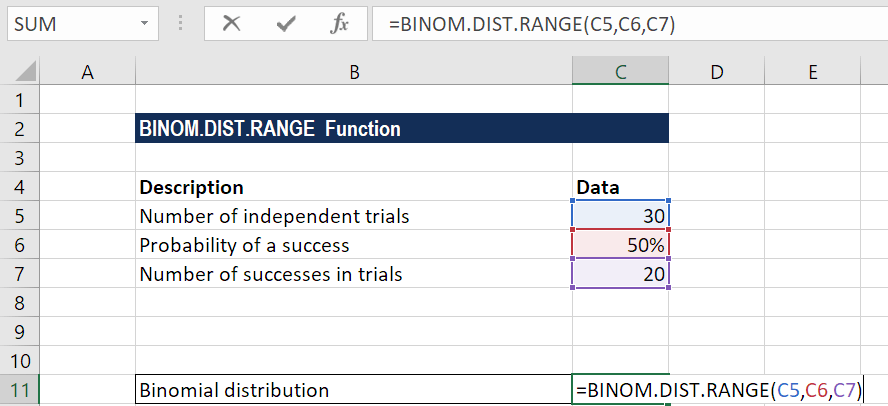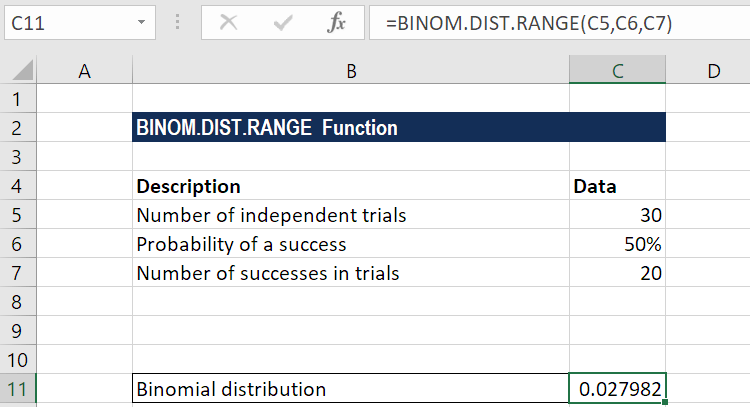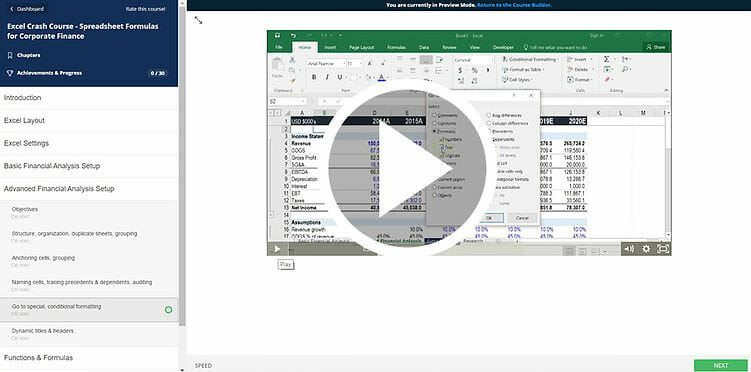# BINOM.DIST.RANGE Function

Calculates the binomial distribution probability for a given number of successes

## What is the BINOM.DIST.RANGE Function?

The BINOM.DIST.RANGE function is categorized under Excel Statistical functions. It will calculate the binomial distribution probability for the number of successes from a specified number of trials falling into a specified range.

In financial analysis, the function can be useful in finding out the probability of, for example, the three bestselling products from a range of products manufactured by a company.

The BINOM.DIST.RANGE function was introduced in MS Excel 2013 and hence is unavailable in earlier versions.

### Formula

=BINOM.DIST.RANGE(trials,probability_s,number_s,[number_s2])

The BINOM.DIST.RANGE function uses the following arguments:

1. Trials (required argument) – This is the number of independent trials. It must be greater than or equal to 0.
2. Probability_s (required argument) – This is the probability of success in each trial. It should be greater than or equal to 0 and less than or equal to 1.
3. Number_s (required argument) – This is the number of successes in trials. It should be greater than or equal to 0. Also, it should be less than or equal to the number of trials.
4. Number_s2 (optional argument) – When provided, it will return the probability that the number of successful trials will fall between number_s and number_s2. It should be greater than or equal to number_s and less than or equal to the number of trials.

### How to use the BINOM.DIST.RANGE Function?

To understand the uses of the BINOM.DIST.RANGE function, let us consider an example:

#### Example

Suppose we are given the following data:The formula for calculating binomial distribution is shown below:We get the result below:### A few notes about the BINOM.DIST.RANGE Function

1. The function will truncate all numerical values to integer.
2. #VALUE! error – Occurs when any of the arguments provided is non-numeric.
3. #NUM! error – Occurs when:
1. The given probability is less than zero or greater than 1.
2. The given number_s is less than zero or greater than the trials argument.
3. The given number_s2 is less than zero or greater than trials or less than number_s.

### Free Excel Course

Work your way toward becoming an expert financial analyst by checking out our Free Excel Crash Course. Learn how to use Excel functions and create sophisticated financial analysis and financial modeling.Thanks for reading CFI’s guide to important Excel functions! By taking the time to learn and master these functions, you’ll significantly speed up your financial analysis. To learn more, check out these additional CFI resources:

• Excel Functions for Finance